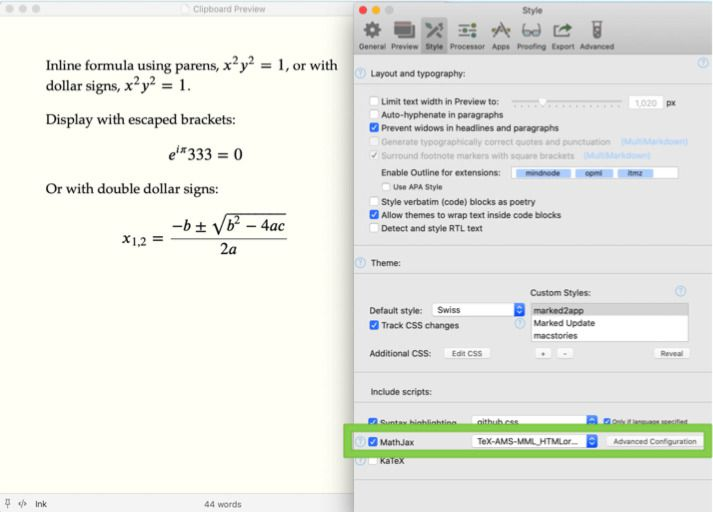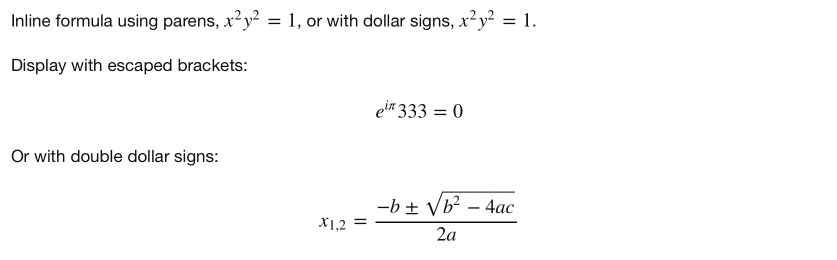← Marked 2 Home

Marked Help

• Full Index
• Changelog

# MathJax

Numbers matter just as much as words.

## Preview formulas with MathJaxBy turning on MathJax in the Marked 2 Preferences, Style pane, the necessary scripts and CSS will be included in the preview. Then MultiMarkdown math syntax can be used in your Markdown document and the results will be displayed.

Example MMD MathJax syntax:

\${e}^{i\pi } 1=0 \$


If you choose to include MathJax in an exported HTML file, a CDN link will be used instead of the embedded MathJax code. This requires an Internet connection to view the rendered MathML.

## Configuration

All of the available configuration presets can be chosen from the dropdown next to the MathJax checkbox. Use these to accomodate various formula styles, LaTeX features, and rendering options.

## MathJax advanced configuration

You can apply additional custom configurations on top of the selected preset by adding a valid JavaScript string in the “Additional MathJax Config” field. This field can contain anything that would normally go inside of a MathJax.Hub.Config() call. For example, the field could contain:

{
"HTML-CSS": {
messageStyle: "normal",
linebreaks: {
automatic: false
}
},
tex2jax: {
inlineMath: [["$","$"],["\$","\$"]],
displayMath: [["$$","$$"],["\$","\$"]],
processEscapes: true
},
TeX: {
Macros: {
tr: "{\\scriptscriptstyle\\mathrm{T}}",
}
}
}


This would turn on MathJax feedback display, disable automatic linebreaks, and define surrounding $ as valid inline equation notation in addition to $equation$, and $$ for display math. (This is an example only,  and $$ are enabled by default.) You can also add LaTeX macros, as shown in the TeX property. With these settings, all of the following renders properly: Inline formula using parens, \${x}^{2} {y}^{2}=1\$, or with dollar signs,${x}^{2} {y}^{2}=1\$.

Display with escaped brackets:

\${e}^{i\pi } 333=0 \label{testme} \$

Or with double dollar signs:

$${x}_{1,2}=\frac{-b\pm \sqrt{{b}^{2}-4ac}}{2a}$$The additional configuration extends the existing object, so only the properties specified will be overridden. Unspecified options will remain at the default for the current preset.

Note that using the MultiMarkdown processor with non-standard delimiters, characters inside the expression are parsed, so symbols like * and ^ will cause typographic changes that will break the MathJax processor. The best solution in these cases is to use the Discount (GFM) processor in Processor preferences.

Marked performs a bit of magic when either MathJax or KaTeX are enabled, converting math syntax to ensure its as compatible as possible with the current processor (MultiMarkdown or Discount). This should be great in all circumstances, but if you find it causing issues, contact support!

## KaTeX

KaTeX is available as an alternative to MathJax. It’s lighter weight and therefore faster to load, which can be great on documents with a large number of formulae. It doesn’t have as many features, though, and some equations that work with MathJax (or LaTeX) may not be supported.

## Automatic Equation Numbering

You can enable equation numbering in Marked 2 Preferences, Style pane. This works with both MathJax and KaTeX. You can select whether numbers appear on the left or right side of the equation.

### In MathJax

In MathJax, this uses the setting:

{
TeX: { equationNumbers: { autoNumber: "all" } }
}


If you want to only number AMS equations, select “AMS only” to the right of the “side” dropdown menu.

### In KaTeX

KaTeX doesn’t offer equation numbering. To simulate this in Marked, CSS is used, and all display equations are numbered.

## Export Issues

Rich Text formats will not handle equations (either by MathJax or KaTeX). They will be hidden in the output document as trying to include the special fonts results in a bigger mess than you’d imagine… This is something I hope to fix at some point, but a shortcoming for right now.